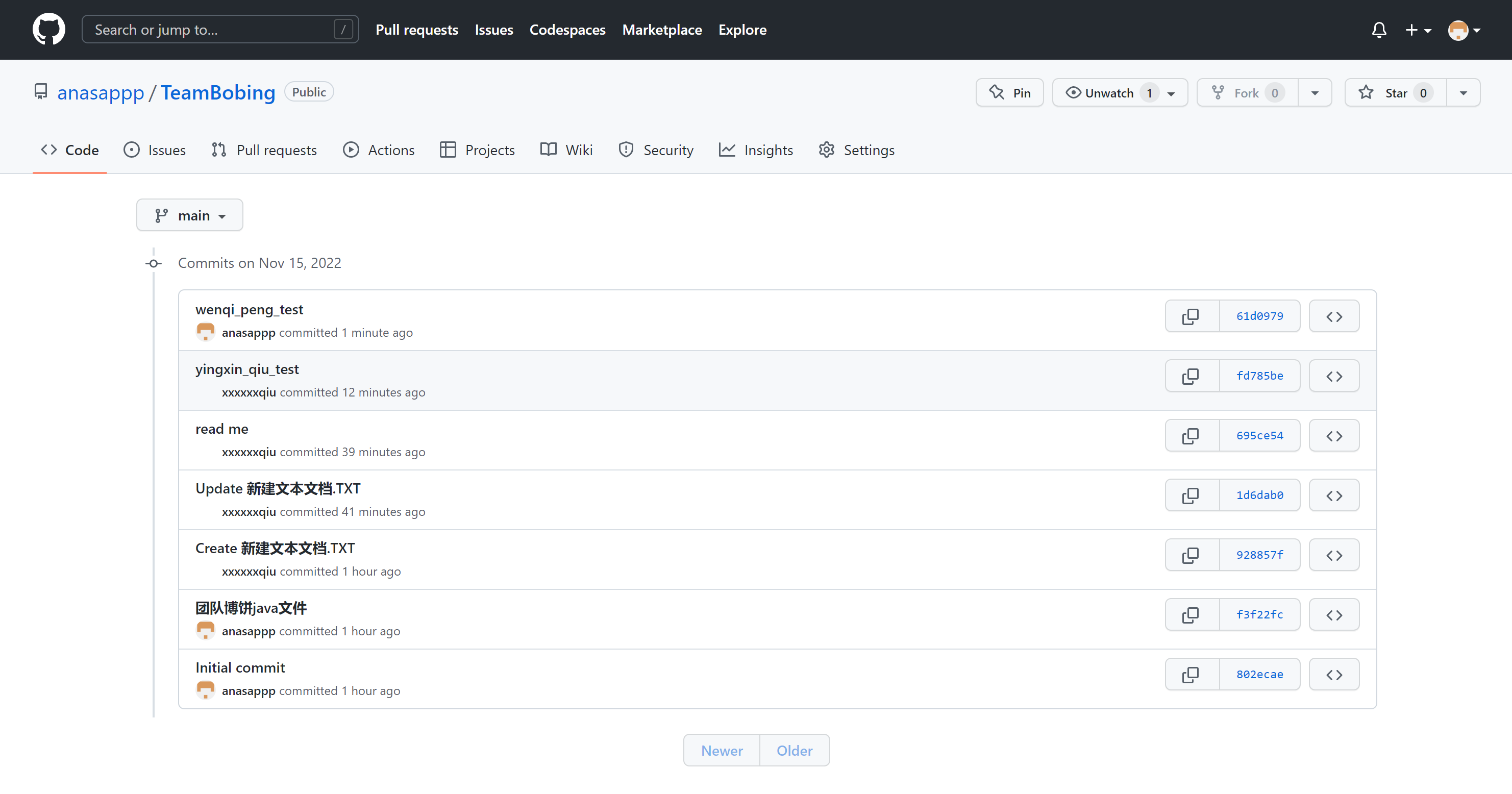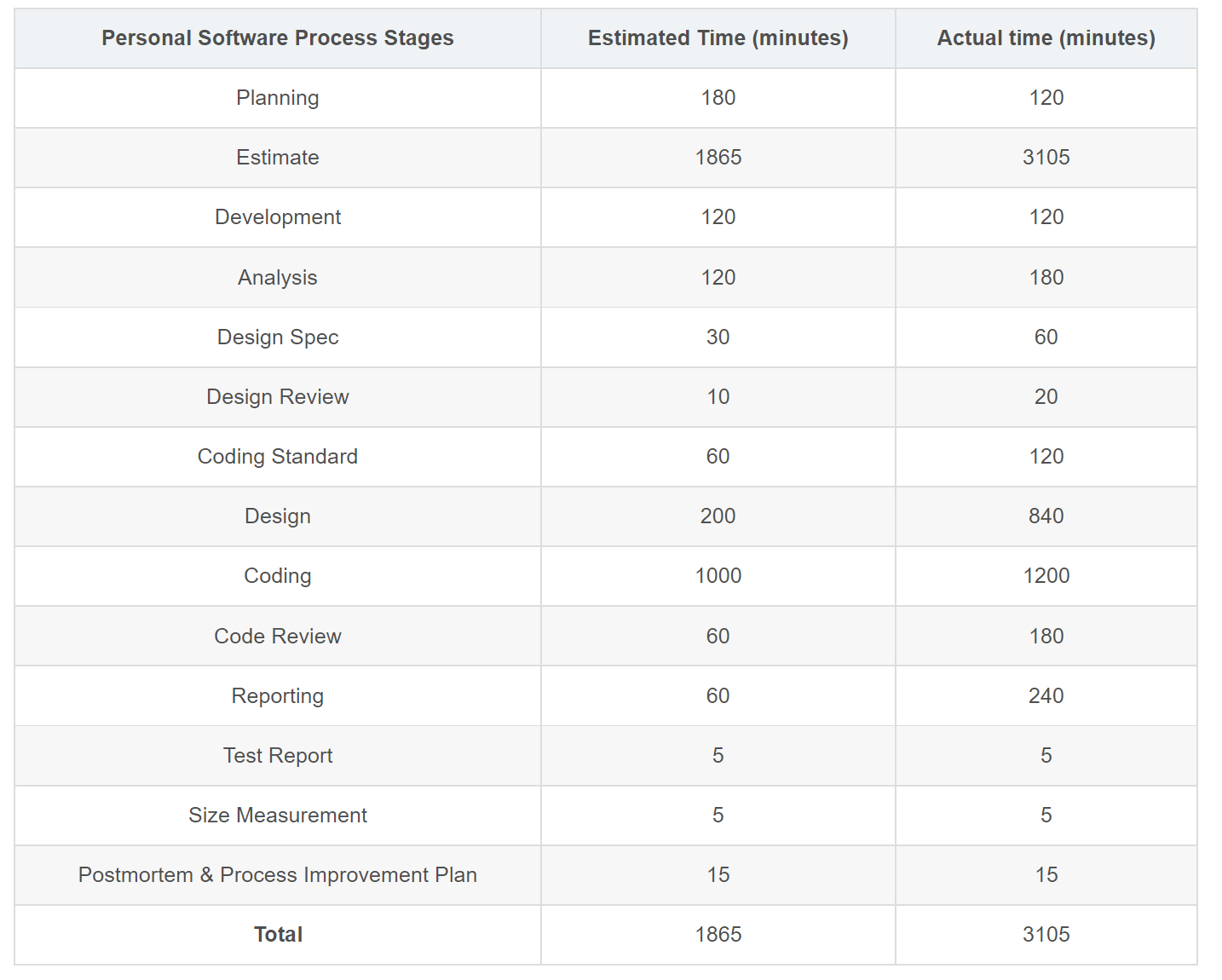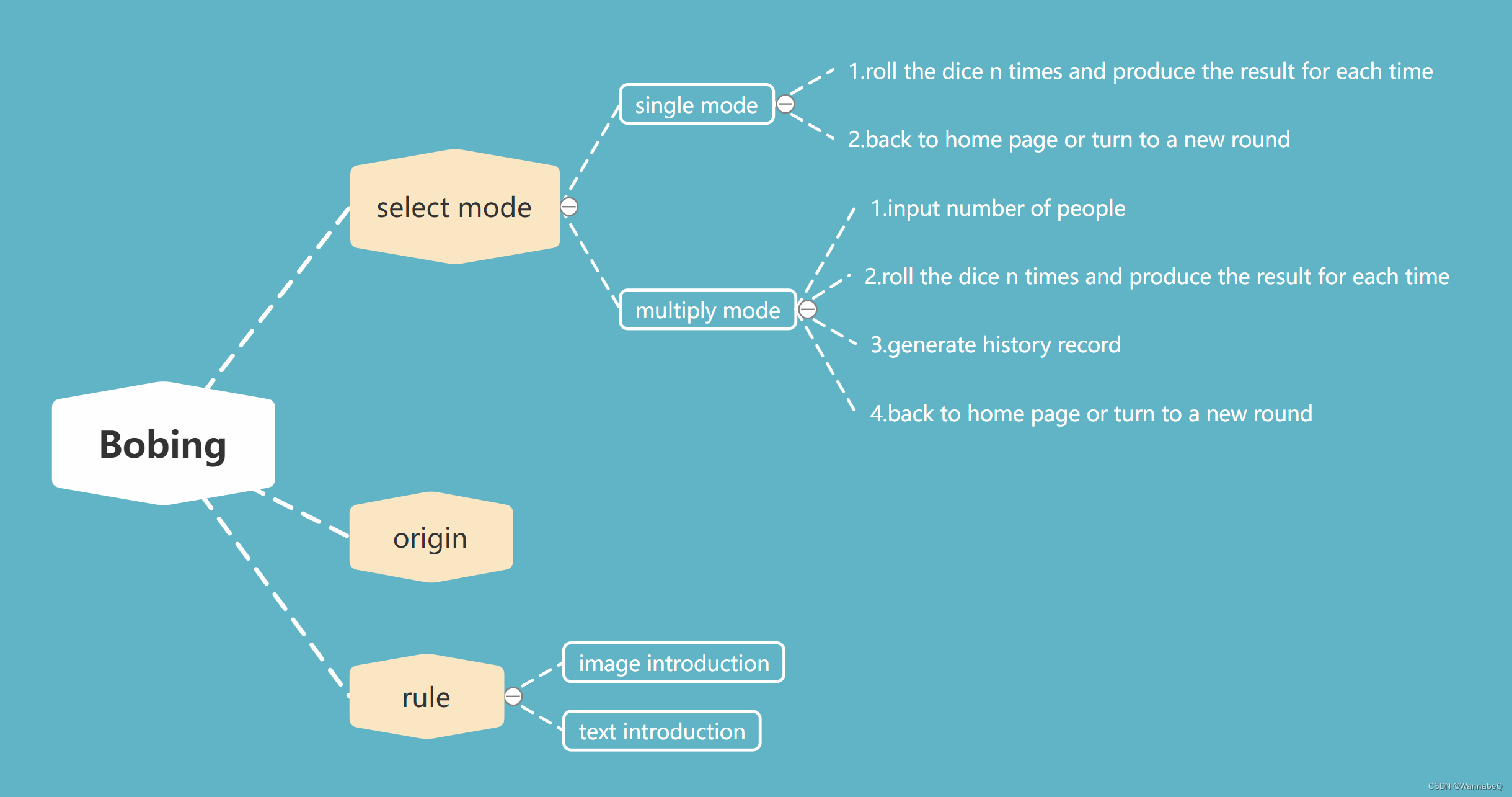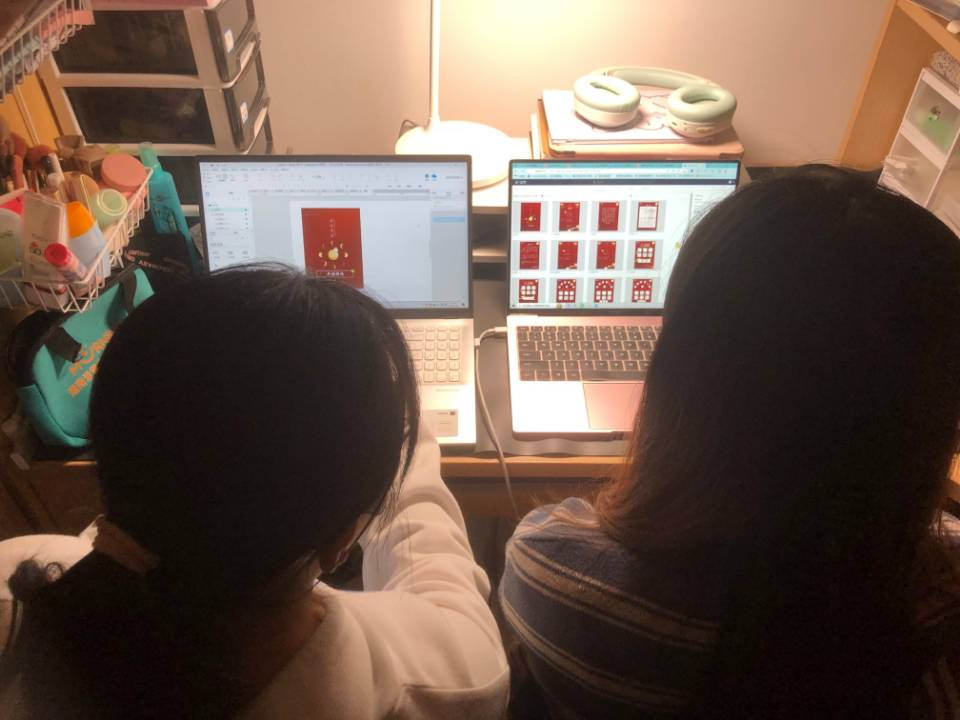# Lab 2-2 The second pair programming assignment

The Link of Requirement of This Assignmenthttps://bbs.csdn.net/topics/608859318
MU STU ID and FZU STU ID20124104_832001205
Teammate's MU STU ID and FZU STU ID20124058_832001227

# Catalog

• Video demo
• Github record & Test
• PSP Form
• The Key or Difficult Functions
• Programming Thinking
• Pair Programming
• Pair programming experience
• What take a long time in coding, arguing, reviewing & Great gains.

### Video demo

https://www.bilibili.com/video/BV1w14y1W7TD/

### Github record & Test### PSP Form### The Key or Difficult Functions

We chose Android Studio to make Bobing software. The most difficult thing is that we have not learned java and AS syntax. Through searching a lot of materials and self-learning, we finally realized the programming of Bobing software. Here's the key code:

1. Intent

This is the most commonly used function in the software, through the Button control, set the click jump event.

Add a Button control and set the onClick function for the button, which is the event that a click occurs. Add the jump command code to the Activity before the jump, then create a second activity.java file, make sure it has an entity class in the existing file, and remember to configure it in AndroidManifest.xml.

``````public class bangyan extends AppCompatActivity {
Intent a, b;

@Override
protected void onCreate(Bundle savedInstanceState) {
super.onCreate(savedInstanceState);
setContentView(R.layout.tanhua);
Button origin = (Button) findViewById(R.id.fanhui);//Call the Button control
origin.setOnClickListener(new ButtonListener());//Set the click jump event for the button
Button rule = (Button) findViewById(R.id.restart);
rule.setOnClickListener(new ButtonListener());

}
class ButtonListener implements View.OnClickListener{
Intent a,b;
@Override
public void onClick(View v) {
switch (v.getId()) {
case R.id.fanhui:
startActivity(a);
break;

case R.id.restart:
startActivity(b);
break;
}
}
}
``````

2. Roll the dice

This is the most basic function of the pie game.

First, the ImageView control of six dice is set in the xml file. Each die needs to call six pictures, that is, one to six dice pictures, and set the position for each control, arranged in two rows.

Now that we have an image of a die, we need to write a java file to roll the die and generate a random result.

Use the Random function to generate six random numbers corresponding to six dice, and connect the random numbers with the corresponding picture resources. The random picture is corresponding to the int value of drawableResource according to the value of rangNumber, and the Drawable class is instanced to set the final picture displayed by the ImageView control.

Now I have six dices that can generate random numbers, and I can match the number of the picture to the random result.

``````//The first die image control
<ImageView
android:id="@+id/dice_image1"
android:layout_width="137dp"
android:layout_height="127dp"
android:layout_alignParentBottom="true"
android:layout_marginStart="7dp"
android:layout_marginLeft="7dp"
android:layout_marginBottom="371dp"
android:layout_toEndOf="@+id/dice_image5"
android:layout_toRightOf="@+id/dice_image5"
android:src="@drawable/empty_dice"
tools:ignore="MissingConstraints" />

``````
``````//                产生随机数
Random rand = new Random();
int randNumber1 = rand.nextInt(6)+1;
//                String randNumber2 = rand.nextInt(6)+1;

int randNumber2 = rand.nextInt(6)+1;
int randNumber3 = rand.nextInt(6)+1;
int randNumber4 = rand.nextInt(6)+1;
int randNumber5 = rand.nextInt(6)+1;
int randNumber6 = rand.nextInt(6)+1;

//                获取对ImageView对象的引用
ImageView diceImage1 = findViewById(R.id.dice_image1);
ImageView diceImage2 = findViewById(R.id.dice_image2);
ImageView diceImage3 = findViewById(R.id.dice_image3);
ImageView diceImage4 = findViewById(R.id.dice_image4);
ImageView diceImage5 = findViewById(R.id.dice_image5);
ImageView diceImage6 = findViewById(R.id.dice_image6);

int drawableResource1,drawableResource2,drawableResource3,
drawableResource4,drawableResource5,drawableResource6;
//                将随机数与对应的图片资源联系起来
switch (randNumber1){
case 1: drawableResource1 = R.drawable.dice_1; break;
case 2: drawableResource1 = R.drawable.dice_2; break;
case 3: drawableResource1 = R.drawable.dice_3; break;
case 4: drawableResource1 = R.drawable.dice_4; break;
case 5: drawableResource1 = R.drawable.dice_5; break;
case 6: drawableResource1 = R.drawable.dice_6; break;
default:
throw new IllegalStateException("Unexpected value: " + randNumber1);
}

//               随机图片根据rangNumber的值对应drawableResource的int值，实例Drawable类
Drawable drawable1 = getBaseContext().getResources().getDrawable(drawableResource1);
//                设置ImageView控件最终显示的图片
diceImage1.setImageDrawable(drawable1);//第一个骰子，之后以此类推
``````

3. Produce result & Single player mode

The number of six dice in the game will produce different results, through the analysis of the rules, I used the counter to achieve, the number of four points in the game is the most important, six four for the number one, a four for the scholar, and so on. The second is the number of twos and ones, which are counted and classified to produce the result.

Now that the single player mode has been implemented, here are the results of the single player mode.``````                //判断奖项
//  存储当前随机数组
int[]  arr;
arr = new int;
arr = randNumber1;
arr = randNumber2;
arr = randNumber3;
arr = randNumber4;
arr = randNumber5;
arr = randNumber6;

Arrays.sort(arr);

int counter = 0;

for(int j=0;j<6;j++){
if (arr[j] == 4){
counter++;
}
}//4出现的个数

//红六勃
if (counter == 6){

Intent intent = new Intent(Multiply.this,hongliubo.class);
startActivity(intent);

}

//插金花
if (counter == 4){
if (arr == 1 && arr == 1){
Intent intent = new Intent(Multiply.this,chajinhua.class);
startActivity(intent);

}
}

int counter1 = 0;//1出现的个数

for(int m=0;m<6;m++){
if (arr[m] == 2){
counter1++;
}
}
//遍地锦（6个1）,榜眼（123456）
if (counter1 == 6){

Intent intent = new Intent(Multiply.this,biandijin.class);
startActivity(intent);

}

if ((arr == 1)&&(arr == 2)&&(arr == 3) && (arr == 4) && (arr == 5)&&(arr==6)){

Intent intent = new Intent(Multiply.this,bangyan.class);
startActivity(intent);

}

//五红(5个4）
if (counter == 5){

Intent intent = new Intent(Multiply.this,wuhong.class);
startActivity(intent);

}
//四红（4个4）
if (counter == 4){
if (arr != 4 && arr != 4){//排除插金花

Intent intent = new Intent(Multiply.this,sihong.class);
startActivity(intent);
}

}
//              探花
if (counter == 3){

Intent intent = new Intent(Multiply.this,tanhua.class);
startActivity(intent);
}
//举人
if (counter == 2){

Intent intent = new Intent(Multiply.this,juren.class);
startActivity(intent);
}
//秀才
if (counter == 1){
if ((arr == 1)&&(arr == 2)&&(arr == 3) && (arr == 4) && (arr == 5)&&(arr==6)){

Intent intent = new Intent(Multiply.this,bangyan.class);
startActivity(intent);
}//排除榜眼
else {

Intent intent = new Intent(Multiply.this,xiucai.class);
startActivity(intent);
}
}

int counter2 = 0;//2出现的个数

for(int j=0;j<6;j++){
if (arr[j] == 2){
counter2++;
}
}

//黑六勃（6个2）
if (counter2 == 6){

Intent intent = new Intent(Multiply.this,heiliubo.class);
startActivity(intent);
}

//五子（5个2）
if (counter2 == 5){

Intent intent = new Intent(Multiply.this,wuzi.class);
startActivity(intent);
}

//进士（4个2）
if (counter2 == 4){

Intent intent = new Intent(Multiply.this,jinshi.class);
startActivity(intent);
}

//未出现4并且2出现的次数小于4并且没有出现6个1则没有中奖
if (counter == 0 && counter2 < 4 && counter1 != 6 ){

Intent intent = new Intent(Multiply.this,wu.class);
startActivity(intent);
}
``````

4. History

In multiplayer we also need to generate a history to record the results of each player.

To do this, we use a two-dimensional dynamic array, where the first column is the number of players, the second column keeps track of each result, and each result is appended to the dynamic array, and finally the array is looped through and printed as a string.

The string is displayed with the TexyView control of AS. First, set the TextView control in the xml file and set the id, and call it in the java file.``````   <TextView
android:id="@+id/array"
android:layout_width="wrap_content"
android:layout_height="wrap_content"
android:layout_centerInParent="true"
android:background="#EBFAF9F9"
android:textColor="#3A3636"
android:textSize="25dp"
android:typeface="serif" />
``````
``````public class Multiply extends AppCompatActivity {
private int N;
private int round=1;
private String[][] list ;

@Override
protected void onCreate(Bundle savedInstanceState) {
super.onCreate(savedInstanceState);
setContentView(R.layout.multi);

N = 5;//用户人数
list = new String[N];
//创建包含数组的list,即可看成是二维数组，储存第几局与每次结果
//固定两行，第一列储存第几次，第二列储存每次结果

//       从布局文件中获取名叫roll_button的按钮对象的引用
Button rollButton = findViewById(R.id.roll_button);
//
//        给按钮rollButton设置点击监听器，一旦用户点击按钮，就触发监听器的onClick方法
rollButton.setOnClickListener(new View.OnClickListener(){
public void onClick(View v){

if (round == 1){
list = "玩家1";
}
if (round == 2){
list = "玩家2";
}
if (round == 3){
list = "玩家3";
}
if (round == 4){
list = "玩家4";
}
if (round == 5){
list = "玩家5";
}
if (round == 6){
list = "玩家6";
}
if (round == 7){
list = "玩家7";
}
if (round == 8){
list = "玩家8";
}
if (round == 9){
list = "玩家9";
}
if (round == 10){
list = "玩家10";
}
if (round == 11){
list = "玩家11";
}
if (round == 12){
list = "玩家12";
}
//                产生随机数
Random rand = new Random();
int randNumber1 = rand.nextInt(6)+1;
//                String randNumber2 = rand.nextInt(6)+1;

int randNumber2 = rand.nextInt(6)+1;
int randNumber3 = rand.nextInt(6)+1;
int randNumber4 = rand.nextInt(6)+1;
int randNumber5 = rand.nextInt(6)+1;
int randNumber6 = rand.nextInt(6)+1;

//                获取对ImageView对象的引用
ImageView diceImage1 = findViewById(R.id.dice_image1);
ImageView diceImage2 = findViewById(R.id.dice_image2);
ImageView diceImage3 = findViewById(R.id.dice_image3);
ImageView diceImage4 = findViewById(R.id.dice_image4);
ImageView diceImage5 = findViewById(R.id.dice_image5);
ImageView diceImage6 = findViewById(R.id.dice_image6);

int drawableResource1,drawableResource2,drawableResource3,
drawableResource4,drawableResource5,drawableResource6;
//                将随机数与对应的图片资源联系起来
switch (randNumber1){
case 1: drawableResource1 = R.drawable.dice_1; break;
case 2: drawableResource1 = R.drawable.dice_2; break;
case 3: drawableResource1 = R.drawable.dice_3; break;
case 4: drawableResource1 = R.drawable.dice_4; break;
case 5: drawableResource1 = R.drawable.dice_5; break;
case 6: drawableResource1 = R.drawable.dice_6; break;
default:
throw new IllegalStateException("Unexpected value: " + randNumber1);
}

//               随机图片根据rangNumber的值对应drawableResource的int值，实例Drawable类
Drawable drawable1 = getBaseContext().getResources().getDrawable(drawableResource1);
//                设置ImageView控件最终显示的图片
diceImage1.setImageDrawable(drawable1);

//              第二个骰子
switch (randNumber2){
case 1: drawableResource2 = R.drawable.dice_1; break;
case 2: drawableResource2 = R.drawable.dice_2; break;
case 3: drawableResource2 = R.drawable.dice_3; break;
case 4: drawableResource2 = R.drawable.dice_4; break;
case 5: drawableResource2 = R.drawable.dice_5; break;
case 6: drawableResource2 = R.drawable.dice_6; break;
default:
throw new IllegalStateException("Unexpected value: " + randNumber2);
}

//                随机图片根据rangNumber的值对应drawableResource的int值，实例Drawable类
Drawable drawable2 = getBaseContext().getResources().getDrawable(drawableResource2);

//                设置ImageView控件最终显示的图片
diceImage2.setImageDrawable(drawable2);

//                第三个骰子

switch (randNumber3){
case 1: drawableResource3 = R.drawable.dice_1; break;
case 2: drawableResource3 = R.drawable.dice_2; break;
case 3: drawableResource3 = R.drawable.dice_3; break;
case 4: drawableResource3 = R.drawable.dice_4; break;
case 5: drawableResource3 = R.drawable.dice_5; break;
case 6: drawableResource3 = R.drawable.dice_6; break;
default:
throw new IllegalStateException("Unexpected value: " + randNumber3);
}
//                随机图片根据rangNumber的值对应drawableResource的int值，实例Drawable类
Drawable drawable3 = getBaseContext().getResources().getDrawable(drawableResource3);
//                设置ImageView控件最终显示的图片
diceImage3.setImageDrawable(drawable3);

//                第四个骰子

switch (randNumber4){
case 1: drawableResource4 = R.drawable.dice_1; break;
case 2: drawableResource4 = R.drawable.dice_2; break;
case 3: drawableResource4 = R.drawable.dice_3; break;
case 4: drawableResource4 = R.drawable.dice_4; break;
case 5: drawableResource4 = R.drawable.dice_5; break;
case 6: drawableResource4 = R.drawable.dice_6; break;
default:
throw new IllegalStateException("Unexpected value: " + randNumber4);
}
//                随机图片根据rangNumber的值对应drawableResource的int值，实例Drawable类
Drawable drawable4 = getBaseContext().getResources().getDrawable(drawableResource4);
//                设置ImageView控件最终显示的图片
diceImage4.setImageDrawable(drawable4);

//                第五个骰子

switch (randNumber5){
case 1: drawableResource5 = R.drawable.dice_1; break;
case 2: drawableResource5 = R.drawable.dice_2; break;
case 3: drawableResource5 = R.drawable.dice_3; break;
case 4: drawableResource5 = R.drawable.dice_4; break;
case 5: drawableResource5 = R.drawable.dice_5; break;
case 6: drawableResource5 = R.drawable.dice_6; break;
default:
throw new IllegalStateException("Unexpected value: " + randNumber5);
}
//                随机图片根据rangNumber的值对应drawableResource的int值，实例Drawable类
Drawable drawable5 = getBaseContext().getResources().getDrawable(drawableResource5);
//                设置ImageView控件最终显示的图片
diceImage5.setImageDrawable(drawable5);

//                第六个骰子

switch (randNumber6){
case 1: drawableResource6 = R.drawable.dice_1; break;
case 2: drawableResource6 = R.drawable.dice_2; break;
case 3: drawableResource6 = R.drawable.dice_3; break;
case 4: drawableResource6 = R.drawable.dice_4; break;
case 5: drawableResource6 = R.drawable.dice_5; break;
case 6: drawableResource6 = R.drawable.dice_6; break;
default:
throw new IllegalStateException("Unexpected value: " + randNumber6);
}
//                随机图片根据rangNumber的值对应drawableResource的int值，实例Drawable类
Drawable drawable6 = getBaseContext().getResources().getDrawable(drawableResource6);
//                设置ImageView控件最终显示的图片
diceImage6.setImageDrawable(drawable6);

//判断奖项
//  存储当前随机数组
int[]  arr;
arr = new int;
arr = randNumber1;
arr = randNumber2;
arr = randNumber3;
arr = randNumber4;
arr = randNumber5;
arr = randNumber6;

Arrays.sort(arr);

int counter = 0;

for(int j=0;j<6;j++){
if (arr[j] == 4){
counter++;
}
}//4出现的个数

//红六勃
if (counter == 6){
list[round-1] = "红六勃";
//                    list[round-1] = 12 + "";
Intent intent = new Intent(Multiply.this,hongliubo.class);
startActivity(intent);

}

//插金花
if (counter == 4){
if (arr == 1 && arr == 1){
list[round-1] = "插金花";
//                        list[round-1] = 13 + "";
Intent intent = new Intent(Multiply.this,chajinhua.class);
startActivity(intent);

}
}

int counter1 = 0;//1出现的个数

for(int m=0;m<6;m++){
if (arr[m] == 2){
counter1++;
}
}
//遍地锦（6个1）,榜眼（123456）
if (counter1 == 6){
list[round-1] = "遍地锦";
//                    list[round-1] = 11 + "";
Intent intent = new Intent(Multiply.this,biandijin.class);
startActivity(intent);

}

if ((arr == 1)&&(arr == 2)&&(arr == 3) && (arr == 4) && (arr == 5)&&(arr==6)){
list[round-1] = "榜眼";
//                    list[round-1] = 6 + "";
Intent intent = new Intent(Multiply.this,bangyan.class);
startActivity(intent);

}

//五红(5个4）
if (counter == 5){
list[round-1] = "五红";
//                    list[round-1] = 9 + "";
Intent intent = new Intent(Multiply.this,wuhong.class);
startActivity(intent);

}
//四红（4个4）
if (counter == 4){
if (arr != 4 && arr != 4){//排除插金花
list[round-1] = "四红";
//                        list[round-1] = 7 + "";
Intent intent = new Intent(Multiply.this,sihong.class);
startActivity(intent);
}

}
//              探花
if (counter == 3){
list[round-1] = "探花";
//                    list[round-1] = 5 + "";
Intent intent = new Intent(Multiply.this,tanhua.class);
startActivity(intent);
}
//举人
if (counter == 2){
list[round-1] = "举人";
//                    list[round-1] = 3 + "";
Intent intent = new Intent(Multiply.this,juren.class);
startActivity(intent);
}
//秀才
if (counter == 1){
if ((arr == 1)&&(arr == 2)&&(arr == 3) && (arr == 4) && (arr == 5)&&(arr==6)){
list[round-1] = "榜眼";
//                        list[round-1] = 6 + "";
Intent intent = new Intent(Multiply.this,bangyan.class);
startActivity(intent);
}//排除榜眼
else {
list[round-1] = "秀才";
//                        list[round-1] = 2 + "";
Intent intent = new Intent(Multiply.this,xiucai.class);
startActivity(intent);
}
}

int counter2 = 0;//2出现的个数

for(int j=0;j<6;j++){
if (arr[j] == 2){
counter2++;
}
}

//黑六勃（6个2）
if (counter2 == 6){
list[round-1] = "黑六勃";
//                    list[round-1] = 10 + "";
Intent intent = new Intent(Multiply.this,heiliubo.class);
startActivity(intent);
}

//五子（5个2）
if (counter2 == 5){
list[round-1] = "五子";
//                    list[round-1] = 8 + "";
Intent intent = new Intent(Multiply.this,wuzi.class);
startActivity(intent);
}

//进士（4个2）
if (counter2 == 4){
list[round-1] = "进士";
//                    list[round-1] = 4 + "";
Intent intent = new Intent(Multiply.this,jinshi.class);
startActivity(intent);
}

//未出现4并且2出现的次数小于4并且没有出现6个1则没有中奖
if (counter == 0 && counter2 < 4 && counter1 != 6 ){
list[round-1] = "无";
//                    list[round-1] = 1 + "";
Intent intent = new Intent(Multiply.this,wu.class);
startActivity(intent);
}

TextView tv = (TextView)findViewById(R.id.array);//获取一个TextView
String ct = "";//定义一个字符串
String s = "历史记录\n";

if (round == N){

for (int m=0;m<N;m++){
for(int j=0;j<2;j++){
ct = ct + list[m][j];//数组拼接成字符串
if (j == 0){
ct = ct +"\u0020\u0020";
}
}
ct = ct + "\n";
}
round = 0;
ct = s +ct;
}

tv.setText(ct);//在TextView中显示数组内容

round = round + 1;
``````

### Programming Thinking

1. Analyze the rules and determine the selection interface, rule interface, origin interface and game interface of the Bocake software, and the game interface needs single mode and multi-player mode.
2. Single-player mode involves rolling dice and producing a result; Multiplayer requires the user to input the number of people and roll the dice multiple times to generate the history.
3. Code skeleton:### Pair Programming### Pair programming experience

Sunday & Monday: Choose programming software, start with Unity firstly, then move to Flutter, set up environments and start learning dart

Tuesday: I quit Flutter and started learning Android Studio and java because my teammate uses Android Studio

Wednesday: Learned the basic rules of ButtonView, TextView, Intent and so on, and realized the button and jump Settings of all pages, completed the production of dice and the generation of random number results

Thursday: Can produce results corresponding to the number of dice, implemented single-player mode programming

Friday: Finished writing the history code, implemented the multiplayer mode programming, and recorded the video

Saturday: Blog

### What take a long time in coding, arguing, reviewing & Great gains.

Event1: Coding software selection
Choose programming software, start with Unity firstly, then move to Flutter, set up environments and start learning dart, I quit Flutter and started learning Android Studio and java because my teammate uses Android Studio

Event2: Dice rolling coding
At first I didn't know how to generate random numbers and map them to corresponding images. After a lot of research, I implemented this function with random function, switch_case statement, and Drawable syntax.

Event3: Code fusion
At the end of the code integration with peers, there are many problems, such as improper naming, version mismatch, not set AndroidManifest configuration, etc., which has taught us a great lesson.

Great Gains
In this team assignment, I realized homemade pancake software, and：

• learned the basic operation of Android Studio
• learned basic knowledge of java
• enhanced the awareness of team communication and teamwork,
• understood the importance of timely communication and making code rules in advance.
...全文
87 回复 打赏 收藏 转发到动态 举报计算机网络实验rdt
Programming Assignment 5: Implementing a Reliable Transport Protocol In this laboratory programming assignment, you will be writing the sending and receiving transport-level code for implementing a simple reliable data transfer protocol. There are two versions of this lab, the Alternating-Bit-Protocol version and the Go-Back-N version. This lab should be fun since your implementation will differ very little from what would be required in a real-world situation.CSE 3100 Systems Programming
CSE 3100 Systems Programming Fall 2019Homework Assignment #1 Due date: 09/12/2019For instructions on how to work on an assignment on Mimir, please review materials in Lab 0. Do not forgetto submi...2021华为 HCIE 数通 LAB 题库 版本 视频 讲解赠笔试题库Programming iOS 12.epub
Programming iOS 12 is actually the second of a pair with my other book, iOS 12 Programming Fundamentals with Swift; it picks up exactly where the other book leaves offDynamic Programming | Set 20 (Maximum Length Chain of Pairs)
Dynamic Programming | Set 20 (Maximum Length Chain of Pairs) You are given n pairs of numbers. In every pair, the first number is always smaller than the second number. A pair (c, d) can foll

286发帖与我相关我的任务• 近7日
• 近30日
• 至今